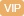| APP | 校园号 | 客服 客服热线：400-863-9889

400-863-9889（2018春•端州区月考）列竖式计算．
699÷3=
430÷6=
728÷9=
【考点】整数的除法及应用【专题】计算题．
【分析】据整数加减乘法和分数加减法的计方进行算．
【解答】解：
4×3=120 00×2=100 0×5=2000
75÷=9…3 200178=2 20×4840
89×0=0 389+40=59 02×3=96
2×4=100 162=32 27÷=3
5-7=58 760=760 103≈500
299×4120 6×+8=50
 3 9
+
 2 9
=
 5 9
 2 7
+
 5 7
=1
1-
 2 3
=
 1 3
 12 12
-
 3 8
+
 1 8
=
 3 4
【点评】口算时注运算号和数据，然后再进一计算．

0/0